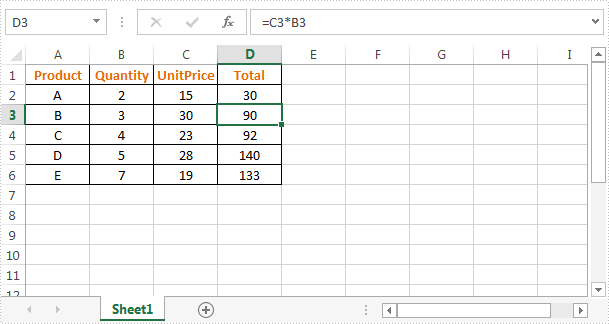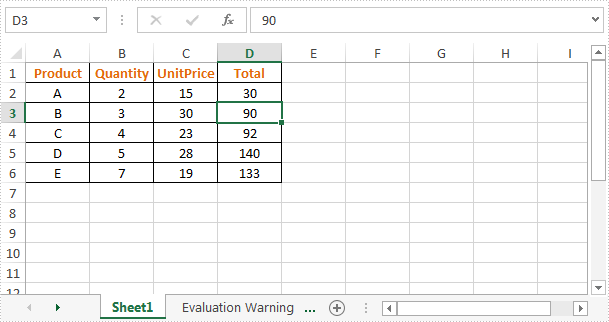Friday, 04 May 2018 06:32

## Remove Formulas from Cells but Keep Values in Excel in C#

Written by  support iceblue
Rate this item

Formulas are commonly used in Excel to calculate data, at some point, we may want to remove these formulas from Excel but keep their calculated values. This article is going to demonstrate how to remove formulas from cells but keep the values using Spire.XLS.

The sample file we used for demonstration:Detail steps:

Step 1: Instantiate a Workbook object and load the excel file.

```Workbook workbook = new Workbook();
```

Step 2: Remove formulas from cells and keep the calculated values.

```//Loop through worksheets
foreach (Worksheet sheet in workbook.Worksheets)
{
//Loop through cells
foreach (CellRange cell in sheet.Range)
{
//If the cell contain formula, get the formula value, clear cell content, and then fill the formula value into the cell
if (cell.HasFormula)
{
Object value = cell.FormulaValue;
cell.Clear(ExcelClearOptions.ClearContent);
cell.Value2 = value;
}
}
}
```

Step 3: Save the file.

```workbook.SaveToFile("DeleteFormula.xlsx", ExcelVersion.Version2013);
```

Screenshot:Full code:

```//Instantiate a Workbook object
Workbook workbook = new Workbook();

//Loop through worksheets
foreach (Worksheet sheet in workbook.Worksheets)
{
//Loop through cells
foreach (CellRange cell in sheet.Range)
{
//If the cell contain formula, get the formula value, clear cell content, and then fill the formula value into the cell
if (cell.HasFormula)
{
Object value = cell.FormulaValue;
cell.Clear(ExcelClearOptions.ClearContent);
cell.Value2 = value;
}
}
}

//Save the file
workbook.SaveToFile("DeleteFormula.xlsx", ExcelVersion.Version2013);
```